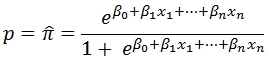# SQL Server LOGIT predicted values function

LOGITPRED

Updated: 27 February 2015

Use the scalar function LOGITPRED to calculate the probability that Y = 1 given a set of co-efficients from a logistic regression and a set of x-values. The probability is estimated as:Syntax

SELECT
[wct].[LOGITPRED](
<@B, nvarchar(max),>
,<@New_x, nvarchar(max),>)

@B
the coefficients from a logit regression. The coefficients are passed in as a string and can either be in the form of a SELECT statement or as the explicit coefficient values separated by commas.
@New_x
the new x-values. The new c-values are passed in as a string and can either be in the form of a SELECT statement or as the explicit x-values to be used in the prediction calculation.

Return Types
float

Remarks
·         You should not pass an x-value for the ß0 coefficient.

Examples
We will run a logistic regression on the following data and then compare the observed y-value to the predicted y-value using the LOGITPROB function. We will use the function to calculate the coefficients.

 Temp Water Male Female 20 0 21 0 21 0 90 6 22 0 91 23 23 0 61 73 24 0 11 41 25 0 4 28 20 1 18 4 21 1 75 9 22 1 68 21 23 1 59 65 24 1 17 46 25 1 7 22

--Put the data into a table
SELECT
*
,male / CAST(male + female as float) as y_obs
INTO
#t
FROM(
VALUES
(20,0,21,0)
,(21,0,90,6)
,(22,0,91,23)
,(23,0,61,73)
,(24,0,11,41)
,(25,0,4,28)
,(20,1,18,4)
,(21,1,75,9)
,(22,1,68,21)
,(23,1,59,65)
,(24,1,17,46)
,(25,1,7,22)
)n(temp,water,male,female)

--Perform the regression
SELECT
*
INTO
#coef
FROM
wct.LOGITSUM('SELECT temp,water,male,female FROM #T',3,4)

--Calculate the predicted y-values and compare
--to the observed y-values
SELECT
t.temp
,t.water
,t.y_obs
,wct.LOGITPRED(
'SELECT
stat_val
FROM
#coef
WHERE stat_name = ''b''',CONCAT(t.temp,',',t.water)) as y_pred
FROM
#t t

This produces the following result.
temp       water                  y_obs                 y_pred
----------- ----------- ---------------------- ----------------------
20           0                      1      0.962666164888472
21           0                 0.9375      0.898550857816897
22           0      0.798245614035088      0.752621841432599
23           0      0.455223880597015      0.511014157141803
24           0      0.211538461538462      0.264148436577182
25           0                  0.125      0.109769453268386
20           1      0.818181818181818      0.961211464524059
21           1      0.892857142857143       0.89487074873382
22           1      0.764044943820225      0.745149498986974
23           1      0.475806451612903      0.501081697117896
24           1       0.26984126984127       0.25649731606484
25           1      0.241379310344828      0.105946142147757

Let's say we wanted to calculate the probability of Y = 1 when temp = 21.5 and water = 0. We can enter any of the following statement to get the result.
SELECT
wct.LOGITPRED(
'SELECT
stat_val
FROM
#coef
WHERE
stat_name = ''b''
ORDER BY
idx ASC',
'21.5,0'
) as p
SELECT
wct.LOGITPRED(
'24.62142607,-1.06858097,-0.039736962',
'21.5,0'
) as p

SELECT
wct.LOGITPRED(
'SELECT 24.62142607,-1.06858097,-0.039736962',
'SELECT 21.5,0'
) as p

SELECT
wct.LOGITPRED(
'24.62142607,-1.06858097,-0.039736962',
'SELECT X FROM (VALUES (0,21.5),(1,0))n(idx,x) ORDER BY idx'
) as p

All of the preceding statements product the following result.
p
----------------------
0.838476405223172

Using the data from Example #2 in the
LOGIT documentation, which used http://www.ats.ucla.edu/stat/data/binary.csv
as input into a table called #mydata, we calculated the the coefficients using the following the SQL which stores the results in table called #mylogit.
SELECT
*
INTO
#mylogit
FROM
wct.LOGIT(
'SELECT
,gre
,gpa
,CASE RANK
WHEN 2 THEN 1
ELSE 0
END
,CASE RANK
WHEN 3 THEN 1
ELSE 0
END
,CASE RANK
WHEN 4 THEN 1
ELSE 0
END
FROM
#mydata'
,1
)
Remember that gre and gpa as treated as continuous data while rank has been treated as discrete data. The possible values for rank are 1, 2, 3, 4.
As in the examples at example at R Data Analysis Examples: Logit Regression which can be found at www.ats.ucla.edu/stat/r/dae/logit.htm we calculate the predicted probability of admission at each value of rank by holding gre and gpa at their means.
SELECT
ROUND(AVG(cast(gre as float)),0) as gre
,ROUND(AVG(cast(gpa as float)),2) as gpa
,x.rank as rank
,ROUND(wct.LOGITPRED(
'SELECT
stat_val
FROM
#mylogit
WHERE
stat_name = ''b'''
,CONCAT(
AVG(cast(gre as float))
,','
,AVG(cast(gpa as float))
,','
,x.rankstr
)
),3) as y_pred
FROM
#mydata
CROSS APPLY(VALUES
(1,'0,0,0'),(2,'1,0,0'),(3,'0,1,0'),(4,'0,0,1')
)x(rank,rankstr)
GROUP BY
x.rank,
x.rankstr

This produces the following result.
gre gpa rank y_pred
--- ---- ---- ------
588 3.39    1 0.517
588 3.39    2 0.352
588 3.39    3 0.219
588 3.39    4 0.185

We can see from the above output that the predicted probability of success is 0.517 when the rank is 1 and 0.185 when the rank is 4 holding gre and gpa at their means.

### SupportCopyright 2008-2023 Westclintech LLC         Privacy Policy        Terms of Service